# 01_clean-housing-data

## Clean housing data¶¶

In this notebook, we download housing data from a popular MLS website, merge, clean, filter and prepare it for subsequent spatial and statistical analysis.

In :
import pandas as pd
import matplotlib.pyplot as plt

%matplotlib inline


### Read data from all CSV files¶¶

In :
csv1_path = 'resources/file1_2018-09-20-14-42-11_4k.csv'
csv2_path = 'resources/file2_2018-09-20-15-04-20_g.csv'
csv3_path = 'resources/file3_2018-09-20-16-02-01_b.csv'


Out:
SALE TYPE SOLD DATE PROPERTY TYPE ADDRESS CITY STATE ZIP PRICE BEDS BATHS STATUS NEXT OPEN HOUSE START TIME NEXT OPEN HOUSE END TIME URL (SEE http://www.redfin.com/buy-a-home/comparative-market-analysis FOR INFO ON PRICING) SOURCE MLS# FAVORITE INTERESTED LATITUDE LONGITUDE
0 MLS Listing NaN Single Family Residential 3445 NE Marine Dr Unit BH04 Portland OR 97211.0 27500.0 0.0 1.0 Active NaN NaN http://www.redfin.com/OR/Portland/3445-NE-Mari… RMLS 18567126 N Y 45.600583 -122.628508
1 MLS Listing NaN Vacant Land NW Thurman St Portland OR 97210.0 30000.0 NaN NaN Active NaN NaN http://www.redfin.com/OR/Portland/NW-Thurman-S… RMLS 18118897 N Y 45.537928 -122.718082
2 MLS Listing NaN Vacant Land NE Rocky Butte Rd Portland OR 97220.0 34777.0 NaN NaN Active NaN NaN http://www.redfin.com/OR/Portland/NE-Rocky-But… RMLS 18454531 N Y 45.542424 -122.565746

3 rows × 27 columns

In :
(prop_df1.shape, prop_df2.shape, prop_df3.shape)

Out:
((3716, 27), (387, 27), (133, 27))

### Merge all CSV into a single sheet¶¶

In :
prop_df = prop_df1.append(prop_df2)
prop_df = prop_df.append(prop_df3)
prop_df.shape

Out:
(4236, 27)

#### Find if there are duplicates¶¶

In :
prop_dup_index = prop_df.duplicated(['MLS#'])
prop_dup = prop_df[prop_dup_index]
prop_dup.shape

Out:
(209, 27)
In :
prop_df.drop_duplicates(subset=['MLS#'], inplace=True)
prop_df.shape

Out:
(4027, 27)

#### Clean column names¶¶

Column names contain illegal characters. Let us rename them

In :
prop_df.columns

Out:
Index(['SALE TYPE', 'SOLD DATE', 'PROPERTY TYPE', 'ADDRESS', 'CITY', 'STATE',
'ZIP', 'PRICE', 'BEDS', 'BATHS', 'LOCATION', 'SQUARE FEET', 'LOT SIZE',
'YEAR BUILT', 'DAYS ON MARKET', '$/SQUARE FEET', 'HOA/MONTH', 'STATUS', 'NEXT OPEN HOUSE START TIME', 'NEXT OPEN HOUSE END TIME', 'URL (SEE http://www.redfin.com/buy-a-home/comparative-market-analysis FOR INFO ON PRICING)', 'SOURCE', 'MLS#', 'FAVORITE', 'INTERESTED', 'LATITUDE', 'LONGITUDE'], dtype='object') In : prop_df.rename(index=str, columns={'$/SQUARE FEET':'PRICE PER SQFT',
'HOA/MONTH':'HOA PER MONTH',
'URL (SEE http://www.redfin.com/buy-a-home/comparative-market-analysis FOR INFO ON PRICING)':'URL',
'MLS#':'MLS'}, inplace=True)
prop_df.columns

Out:
Index(['SALE TYPE', 'SOLD DATE', 'PROPERTY TYPE', 'ADDRESS', 'CITY', 'STATE',
'ZIP', 'PRICE', 'BEDS', 'BATHS', 'LOCATION', 'SQUARE FEET', 'LOT SIZE',
'YEAR BUILT', 'DAYS ON MARKET', 'PRICE PER SQFT', 'HOA PER MONTH',
'STATUS', 'NEXT OPEN HOUSE START TIME', 'NEXT OPEN HOUSE END TIME',
'URL', 'SOURCE', 'MLS', 'FAVORITE', 'INTERESTED', 'LATITUDE',
'LONGITUDE'],
dtype='object')

#### Drop unnecessary columns¶¶

While it is beneficial to have a lot of features (columns) in our dataset, it is also important to drop those that are highly correlated to an existing field or a derivative of one or fields that we will never use. In the following, we drop fields that we will never use in this analysis.

In :
prop_df.drop(columns=['SOLD DATE','NEXT OPEN HOUSE START TIME', 'NEXT OPEN HOUSE END TIME',
'FAVORITE', 'INTERESTED'], inplace=True)

In :
prop_df.columns

Out:
Index(['SALE TYPE', 'PROPERTY TYPE', 'ADDRESS', 'CITY', 'STATE', 'ZIP',
'PRICE', 'BEDS', 'BATHS', 'LOCATION', 'SQUARE FEET', 'LOT SIZE',
'YEAR BUILT', 'DAYS ON MARKET', 'PRICE PER SQFT', 'HOA PER MONTH',
'STATUS', 'URL', 'SOURCE', 'MLS', 'LATITUDE', 'LONGITUDE'],
dtype='object')

#### Find and fill missing values¶¶

Missing values in certain columns are critical - beds, bath, price, latitude, longitude. We will drop these rows. Missing values in remaining columns are not so critical, we will fill them with 0 or average values.

Before we fill, let us explore the histograms of numerical columns. With pandas, this can be accomplished with a single command as shown below:

In :
# explore distribution of numeric columns
ax_list = prop_df.hist(bins=25, layout=(4,4), figsize=(15,15))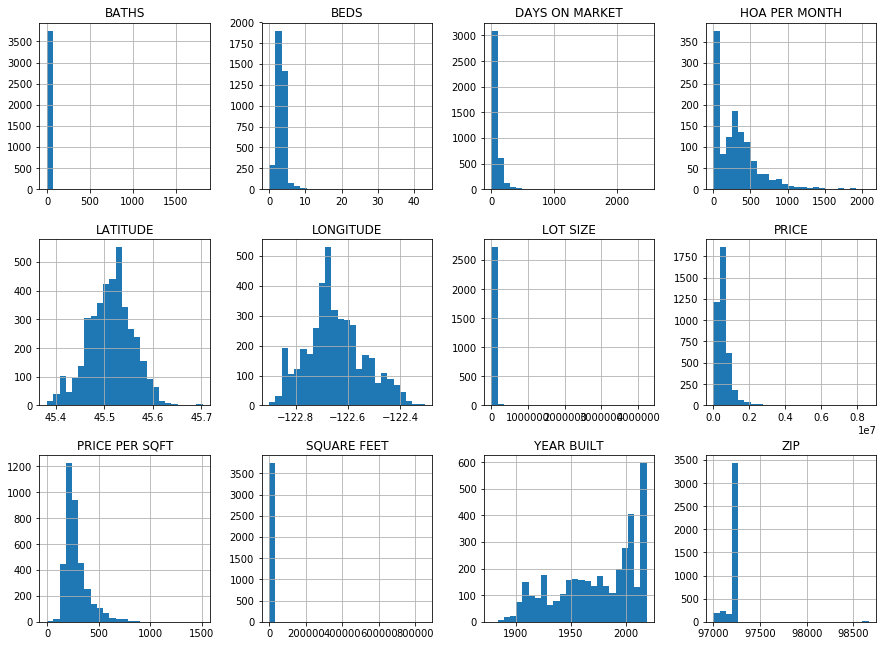In :
# drop rows with missing values in critical columns
prop_df_nona = prop_df.dropna(axis=0, how='any', # if any of these cols are empty, remove row
subset=['BEDS','BATHS', 'PRICE', 'YEAR BUILT', 'LATITUDE','LONGITUDE'])
prop_df_nona.shape

Out:
(3653, 22)

Let us impute for missing values using different strategies for different columns.

In :
prop_df_nona['HOA PER MONTH'].fillna(value=0, inplace=True)
prop_df_nona['LOT SIZE'].fillna(value=prop_df_nona['LOT SIZE'].median(), inplace=True)
prop_df_nona['PRICE PER SQFT'].fillna(value=prop_df_nona['PRICE PER SQFT'].median(), inplace=True)
prop_df_nona['SQUARE FEET'].fillna(value=prop_df_nona['SQUARE FEET'].median(), inplace=True)
prop_df_nona['YEAR BUILT'].fillna(value=prop_df_nona['YEAR BUILT'].mode(), inplace=True)
prop_df_nona['ZIP'].fillna(value=prop_df_nona['SQUARE FEET'].mode(), inplace=True)

/Users/atma6951/anaconda3/envs/geosaurus_gold/lib/python3.6/site-packages/pandas/core/generic.py:5434: SettingWithCopyWarning:
A value is trying to be set on a copy of a slice from a DataFrame

See the caveats in the documentation: http://pandas.pydata.org/pandas-docs/stable/indexing.html#indexing-view-versus-copy
self._update_inplace(new_data)

In :
# explore distribution of numeric columns
ax_list = prop_df_nona.hist(bins=25, layout=(4,4), figsize=(15,15))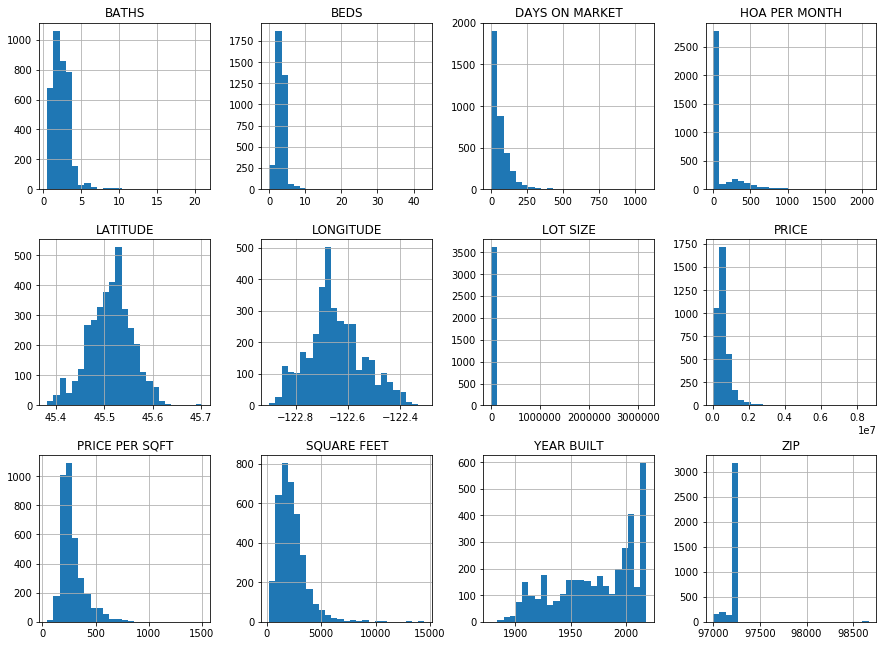### Explore distribution of numeric columns¶¶

As can be seen from histogram, some numeric columns are heavily sqewed by outliers. Let us pull up statistics for each of these columns

In :
prop_df_nona.describe().round(3)

Out:
ZIP PRICE BEDS BATHS SQUARE FEET LOT SIZE YEAR BUILT DAYS ON MARKET PRICE PER SQFT HOA PER MONTH LATITUDE LONGITUDE
count 3653.000 3653.000 3653.000 3653.000 3653.000 3653.000 3653.000 3653.000 3653.000 3653.000 3653.000 3653.000
mean 97210.091 609851.635 3.316 2.428 2291.079 13448.155 1973.970 62.268 281.446 105.865 45.513 -122.648
std 93.873 458104.003 1.762 1.340 1342.049 72390.700 36.279 72.488 118.235 223.111 0.046 0.107
min 97002.000 35000.000 0.000 0.500 212.000 25.000 1878.000 1.000 44.000 0.000 45.382 -122.902
25% 97206.000 359900.000 2.000 2.000 1400.000 5227.000 1948.000 15.000 206.000 0.000 45.483 -122.712
50% 97217.000 495000.000 3.000 2.500 2062.000 6969.000 1980.000 42.000 251.000 0.000 45.516 -122.661
75% 97229.000 699934.000 4.000 3.000 2862.000 8712.000 2006.000 84.000 321.000 74.000 45.541 -122.584
max 98664.000 8650000.000 43.000 21.000 14500.000 3167247.000 2019.000 1080.000 1505.000 2091.000 45.703 -122.306

#### Build statistical filters to remove outliers¶¶

In this segment, we build $6\sigma$ and Inter Quartile Range (IQR) filters to remove outliers from our dataset.

##### 6 sigma filter¶¶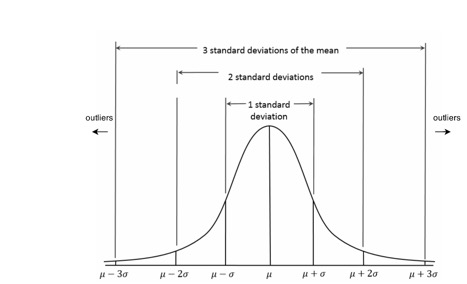Statistically, about 99.5% of data falls within $\pm 3 \sigma$ from $\mu$ (mean). Thus, for fairly normally distributed data, $6\sigma$ filter yields a good resultant dataset.

##### IQR filter¶¶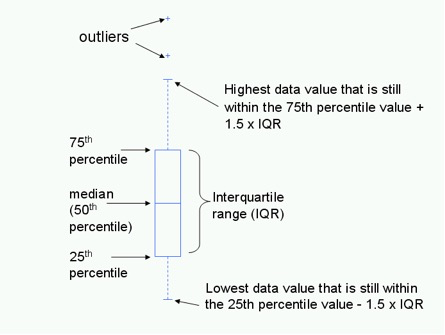Howerver, certain columns such as BEDS, LOT SIZE are heavily sqewed. For such, the Inter Quartile Range filter (which uses median as a measure of centrality unlike 6sigma which uses mean) yields a robust filter as it is unaffected by outliers like the 6 sigma filter.

In :
def six_sigma_filter(df, column):
sigma = df[column].std()
mu = df[column].mean()
three_sigma = [mu-(3*sigma), mu+(3*sigma)]
print("Column:{}, Mean:{}, Sigma:{}, 3sigma_range: {}:{}".format(column,mu.round(3),
sigma.round(3),
three_sigma.round(2),
three_sigma.round(2)))

# filter
df_to_keep = df[(df[column] > three_sigma) & (df[column] < three_sigma)]

# prints
num_rows_dropped = prop_df.shape - df_to_keep.shape
print("Number of rows dropped: " + str(num_rows_dropped))

return df_to_keep

In :
def iqr_filter(df, column):
med = df[column].median()
p_25 = df[column].quantile(q=0.25)
p_75 = df[column].quantile(q=0.75)

# find valid range
iqr_range = [med-(2*p_25), med+(2*p_75)]
print("Column: {}, Median:{}, 25%:{}, 75%:{}, IQR:{}:{}".format(column,med,
p_25,p_75,
iqr_range.round(2),
iqr_range.round(2)))

# filter
df_to_keep = df[(df[column] > iqr_range) & (df[column] < iqr_range)]

#prints
num_rows_dropped = prop_df.shape - df_to_keep.shape
print("Number of rows dropped: " + str(num_rows_dropped))

return df_to_keep


#### Filter columns using both filters and compare¶¶

In :
prop_df2 = six_sigma_filter(prop_df_nona, 'BATHS')

Column:BATHS, Mean:2.428, Sigma:1.34, 3sigma_range: -1.59:6.45
Number of rows dropped: 422

In :
prop_df2_iqr = iqr_filter(prop_df_nona, 'BATHS')

Column: BATHS, Median:2.5, 25%:2.0, 75%:3.0, IQR:-1.5:8.5
Number of rows dropped: 396

In :
prop_df4 = six_sigma_filter(prop_df2, 'BEDS')

Column:BEDS, Mean:3.206, Sigma:1.263, 3sigma_range: -0.58:7.0
Number of rows dropped: 459

In :
prop_df4_iqr = iqr_filter(prop_df2_iqr, 'BEDS')

Column: BEDS, Median:3.0, 25%:2.0, 75%:4.0, IQR:-1.0:11.0
Number of rows dropped: 403

In :
(prop_df4.shape, prop_df4_iqr.shape)

Out:
((3539, 22), (3624, 22))
In :
ax_list = prop_df4.hist(bins=25, layout=(4,4), figsize=(15,15))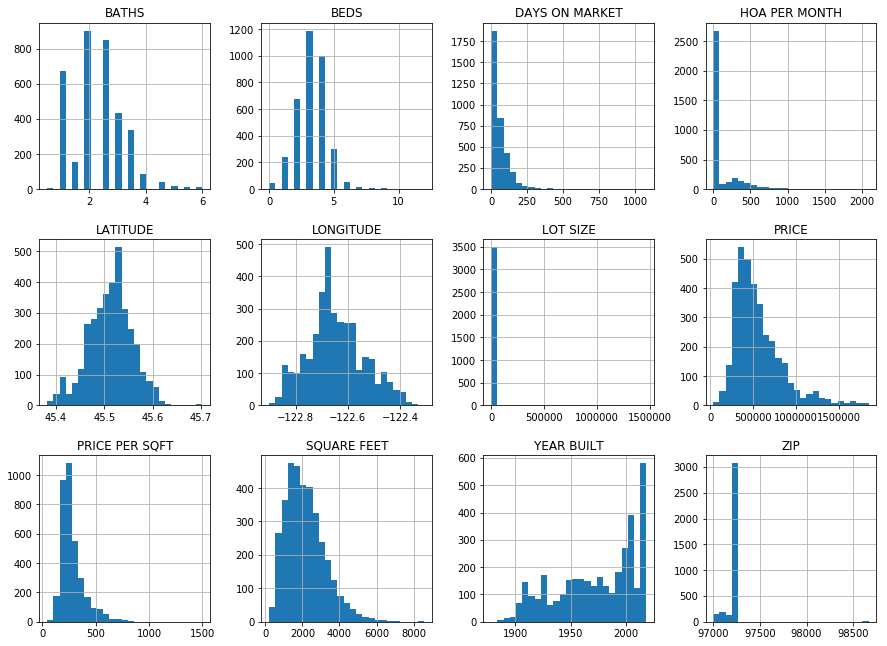In :
ax_list = prop_df4_iqr.hist(bins=25, layout=(4,4), figsize=(15,15))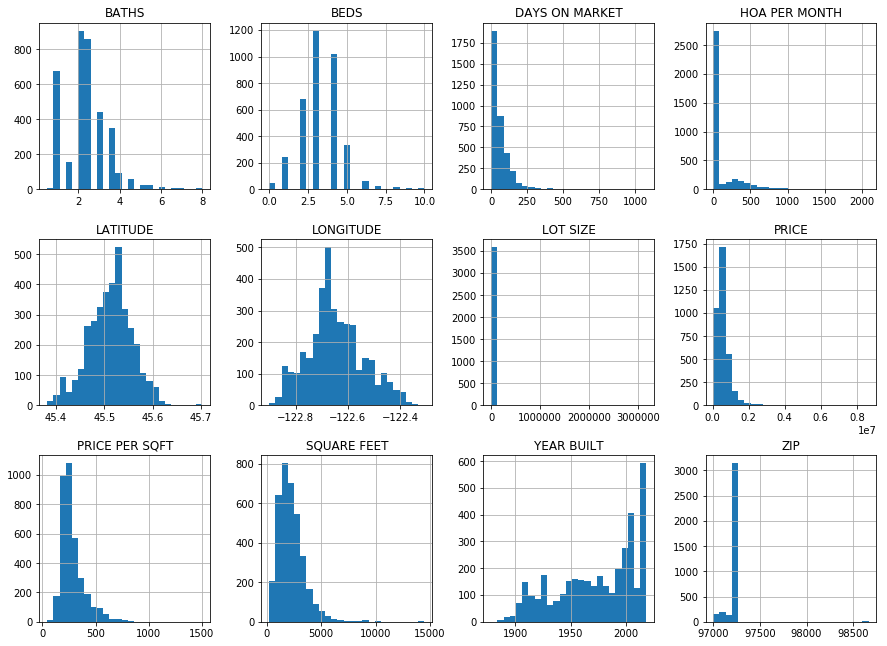### Write cleaned, appended table to disk¶¶

The IQR filter yields a better result in our case as the resulting histograms of numeric columns show a nice normal distribution. We proceed with this dataset and write that to disk.

In :
prop_df4_iqr.to_csv('resources/houses_for_sale_filtered.csv')